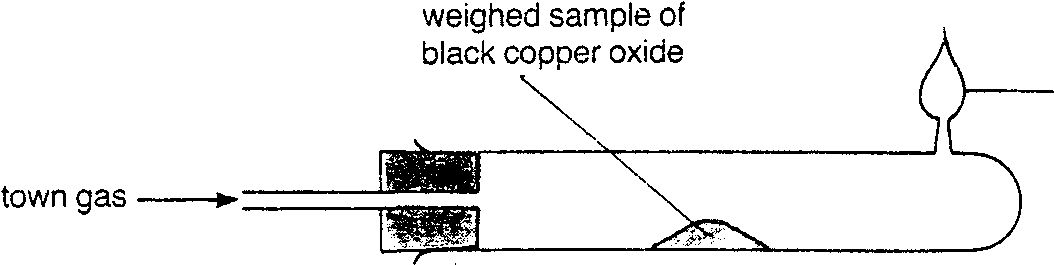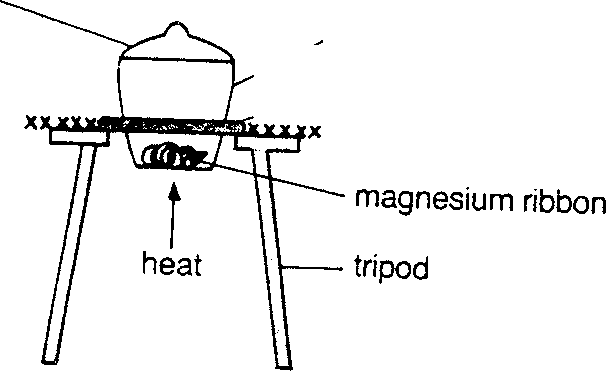## Waying.edu.hkEmpirical and molecular formulae
A. Introduction
Formula in Chemistry is very important to simplify the presentation of substances. There are many types of formula, for example, chemical formula, molecular formula, structural formula etc. Empirical formula of a compound shows the simplest whole-number ratio of atoms or ions
present. It does NOT represent the actual number of atoms bonded together.
Molecular formula of a compound shows the actual number of each kind of atoms present
in one molecule (for covalent compounds only). How about ionic compounds?
The major different between empirical and molecular formula is that the former may not exit as actual substance. For example, the empirical formula of C2H4 (ethene) is CH2 but it does not exist. Determining chemical formulae by experiments
To determine the empirical formula of black copper(II) oxide mass of test-tube + black copper(II) oxide = 22.950g mass of test-tube + copper Thus the empirical formula of black copper(II) oxide is __________.To determine the empirical formula of magnesium oxide mass of crucible + lid + magnesium oxide Oxygen (O)
Thus the empirical formula of magnesium oxide is __________. Examples of finding empirical formulae
Example 1: Hydrocarbon M contains 85.7% carbon. Calculate the empirical formula of M. Answer: percentage by mass of carbon = 85.7
percentage by mass of hydrogen = 100 - 85.7 = 14.3
Assume we have 100g of M,
Hydrogen (H)
The empirical formula of M is ________. Aspirin contains 60% carbon, 4.5% of hydrogen and 35.5% oxygen. What is the empirical formula? Assume we have 100g of aspirin, then there is 60g of carbon, 4.5g of
hydrogen, 35.5g of oxygen.
Oxygen (O)
Thus the empirical formula for aspirin is __________. The oxide of metal M (relative atomic mass = 52) contains 31.6% by mass of oxygen. What is the empirical formula? 2.8g of X is completely reacted with excess Y to form 17g of compound. What is the empirical formula of the compound? (Relative atomic masses of X = 28, Y = 35.5). Examples of finding molecular formulae
Example 1: 1.5g hydrocarbon M contains 85.7% carbon is found to occupy 600cm3 at s.t.p. Calculate the empirical and molecular formula of M. (molar volume of M at s.t.p. = 22.4dm3) percentage by mass of carbon = 85.7
percentage by mass of hydrogen = 100 - 85.7 = 14.3
Assume we have l00g of M,
Thus the molecular formula of M is __________. A compound L contains 52.18% carbon, 13.04% hydrogen and 34.78% oxygen by mass. 9.2g of L are found to occupy 4,480cm3 at s.t.p. What is the molecular formula of L? Compound Q contains 69.8% carbon, 11.7% It, and some oxygen. 560cm3 of its vapor weighs 2.15g at s.t.p. a. Calculate the relative molecular mass of Q. Molecular formulae of simple organic molecules
26cm3 of a gaseous hydrocarbon required 91cm3 of oxygen for complete combustion and gave 52cm3 of carbon dioxide. Find the formula of the hydrocarbon. let the molecular formula be CxHy 26cm3 of CxHy require 91 cm3 of oxygen to give 52cm3 of carbon dioxide 1 cm3 of CxHy, requires 3.5cm3 of oxygen to give 2cm3 of carbon dioxide consider the equation: CxHy(g) + O2(g) Æ CO2(g) + H2O(l) therefore molecular formula is ________________. 17cm3 of a compound of carbon, hydrogen and oxygen required 68cm3 of oxygen for complete combustion. The experiment was maintained at a temperature of 120°C and the volume of carbon dioxide and steam formed were 51 cm3 each. What is the formula of the original compound? Determine molecular formulae from combustion data Example 1: Upon complete combustion of 0.46g of a compound containing carbon, hydrogen and oxygen, 0.88g of carbon dioxide and 0.54g of water were formed. Calculate the empirical formula of the original compound. 0.88g of CO2 contain _______ fraction of carbon = _________ g 0.54g of H2O contain _______ fraction of hydrogen = __________ g mass of oxygen = ______________________________ The empirical formula is ________________. A compound contains carbon, oxygen and hydrogen only. 4.5g of it when completely burnt in oxygen, gives 4.4g of carbon dioxide and 0.9g of water. Its molecular mass is found to be 90. Find the molecular formula. A gaseous hydrocarbon with vapor density equals 21 was burnt completely in air. The gaseous products were first passed through on U-tube (cooling under water) then to another test tube containing potassium hydroxide solution. The results of the experiment were recorded as below: weight of U-tube before experiment weight of test-tube & content before experiment weight of test-tube & content after experiment What was the function of potassium hydroxide solution? Find the empirical and molecular formulae of the hydrocarbon. (Hint : 2 x vapor density of a gas = relative molecular mass of the gas)

Source: http://www.waying.edu.hk/chemistry/Learning_materials_for_AL_Chemistry/notes%5Cempirical%20and%20molecular%20formulae.pdf

### inclusiveeurope.se

Midlife - The Free Theatre "Stefan" has a bit much at home and at work. Manager "Eve" is trying to get him to work even more. The Free Theatre wants, with their performance Midlife, to get people to discuss important issues. In the middle of the murmur a voice is heard:  - Someone forgot a book here: Black belt in everyday effectiveness! She who asks is Susanne Olofsson,

### charette.corg.umontreal.ca

pKa Values For complex chelating agents, see also reference 77. Note. This document was compiled by W.P. Jencks and has been added to by F.H. Westheimer Compound * Indicates a thermodynamic value. PHOSPHATES AND PHOSPHONATES Phosphates Compound Arylphosphonic acids Phosphonates H2O3PCH2CH(CH3)PO3H2 <2, 2.6, 7.00, 9.27 57**These values were obtained in 50% ethanol.